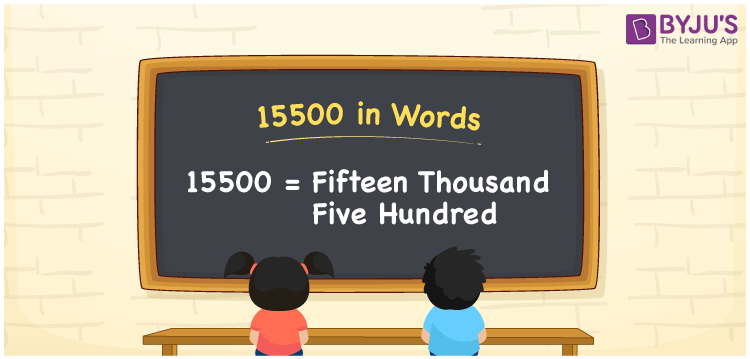# 15500 in Words

15500 in words is written as Fifteen Thousand Five Hundred. In both the International System of Numerals and the Indian System of Numerals, 15500 is written as Fifteen Thousand Five Hundred. The number 15500 is a Cardinal Number as it could represent some quantity. For example, “that mobile phone costs 15500 rupees”.

 15500 in words Fifteen Thousand Five Hundred Fifteen Thousand Five Hundred in Number 15500

## 15500 in English Words

15500 in English words is read as “Fifteen Thousand Five Hundred”.## How to Write 15500 in Words?

To write 15500 in words, we shall use the place value chart. In the place value chart, put 1 in the ten thousands place, 5 in the thousands and hundreds place and 0 in the rest. Let us make a place value chart to write the number 15500 in words.

 Ten Thousands Thousands Hundreds Tens Ones 1 5 5 0 0

Thus, we can write the expanded form as:

1 × Ten Thousand + 5 × Thousand + 5 × Hundred + 0 × Ten + 0 × One

= 1 × 10000 + 5 × 1000 + 5 × 100 + 0 × 10 + 0 × 1

= 10000 + 5000 + 500 + 0 + 0

= 15500

= Fifteen Thousand Five Hundred

15500 is a natural number, the successor of 15499 and the predecessor of 15501.

15500 in words – Fifteen Thousand Five Hundred

• Is 15500 an odd number? – No
• Is 15500 an even number? – Yes
• Is 15500 a perfect square number? – No
• Is 15500 a perfect cube number? – No
• Is 15500 a prime number? – No
• Is 15500 a composite number? – Yes

## Frequently Asked Questions (FAQs) on 15500 in Words

Q1

### How to write 15500 in words?

15500 in words is written as Fifteen Thousand Five Hundred.
Q2

### How to write 15500 in the International and Indian System of Numerals?

In both, the system of numerals, 15500 in words, is written as Fifteen Thousand Five Hundred.
Q3

### How to write 15500 in a place value chart?

In the place value chart, write 1 in the ten thousands, 5 in the thousands and the hundreds, and 0 in the tens and ones, respectively.
Q4

### Is 15500 an Even Number?

Yes. 15500 is an even number.
Q5

### Is 15500 a Perfect Square Number?

No.15500 is not a perfect square number.